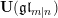Nagoya Mathematical Journal ( IF 0.556 ) Pub Date : 2018-04-30 , DOI: 10.1017/nmj.2018.12
JIE DU; HAIXIA GU; ZHONGGUO ZHOU

We investigate products of certain double cosets for the symmetric group and use the findings to derive some multiplication formulas for the$q$ -Schur superalgebras. This gives a combinatorialization of the relative norm approach developed in Du and Gu (A realization of the quantum supergroup$\mathbf{U}(\mathfrak{g}\mathfrak{l}_{m|n})$ , J. Algebra 404 (2014), 60–99). We then give several applications of the multiplication formulas, including the matrix representation of the regular representation and a semisimplicity criterion for$q$ -Schur superalgebras. We also construct infinitesimal and little$q$ -Schur superalgebras directly from the multiplication formulas and develop their semisimplicity criteria.

down
wechat
bug